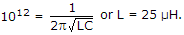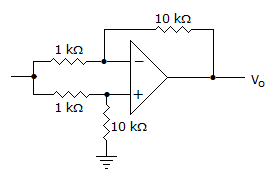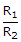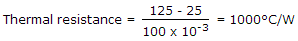# Electronics and Communication Engineering - Analog Electronics

21.

A negative feedback can be of

 A. 2 types B. 3 types C. 4 types D. only 1 type

Explanation:

Series voltage, series current, shunt voltage and shunt current.

22.

In a class C power amplifier the input frequency of ac signal is 1 MHz. If tank circuit has C = 1000 pF, the value of L =

 A. 25 μH B. 50 μH C. 100 μH D. 200 μH

Explanation:23.

The Vo of the op-amp circuit shown in the given isA. 11 Vi B. 10 Vi C. Vi D. zero

Explanation:

V0 =(Vs2 - Vs1)[Vs1 - Vs1] = 0.

24.

In calculating output impedance of an amplifier the source is replaced by an open circuit.

 A. True B. False

Explanation:

It is replaced by short circuit.

25.

For transistor 2 N 338 the manufacturer specifies Pmax = 100 mW at 250°C free air temperature and maximum junction temperature of 125°C. Its thermal resistance is

 A. 10° C/W B. 100° C/W C. 1000° C/W D. 5000° C/W.